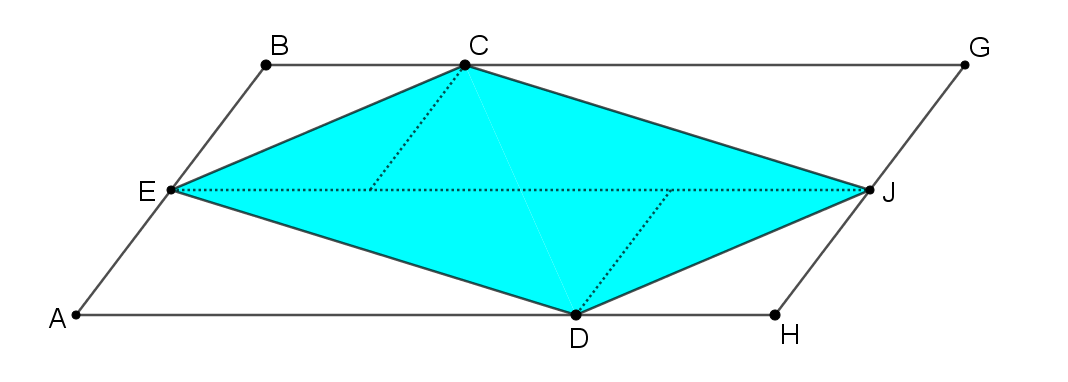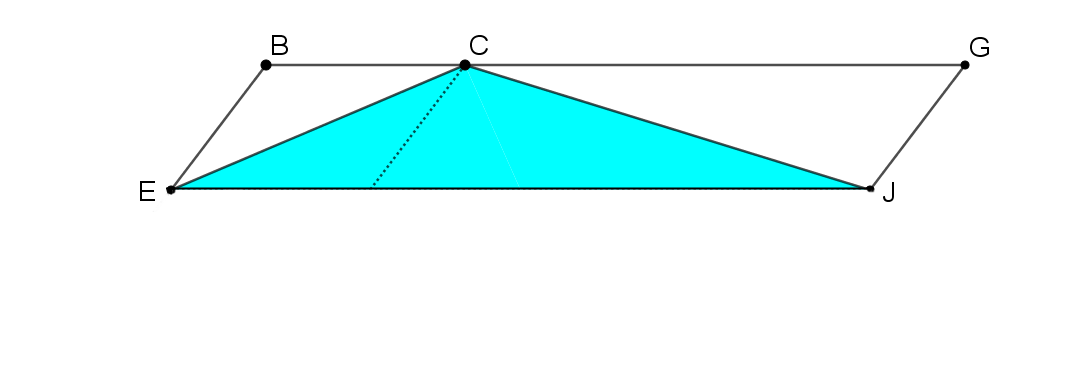#### You may also like### Fitting In

The largest square which fits into a circle is ABCD and EFGH is a square with G and H on the line CD and E and F on the circumference of the circle. Show that AB = 5EF. Similarly the largest equilateral triangle which fits into a circle is LMN and PQR is an equilateral triangle with P and Q on the line LM and R on the circumference of the circle. Show that LM = 3PQ### Look Before You Leap

Can you spot a cunning way to work out the missing length?### Triangle Midpoints

You are only given the three midpoints of the sides of a triangle. How can you construct the original triangle?

# Triangle in a Trapezium

##### Age 11 to 16Challenge Level

You may find it useful to print off some dotty paper or use the dotty grids environment to explore the first part of this problem.

In the two trapeziums below, we have drawn triangles by joining the vertices of one of the non-parallel sides to the midpoint of the opposite side.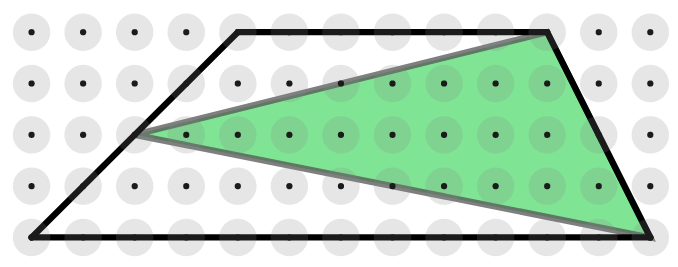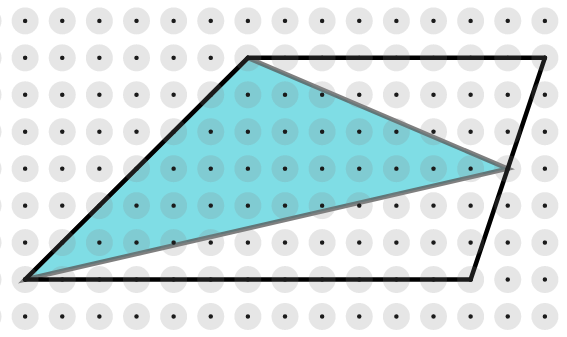Is there a relationship between the area of each trapezium and the area of the shaded triangle contained within it?

Draw some more trapeziums and construct triangles inside them in the same way. Does the same relationship hold?

Can you find a way to prove that your relationship will always hold for any trapezium?

Once you have had a go at proving it, click below to see some possible starting points.

Can you take each starting point and develop it into a full proof?

Starting Point 1

In this diagram, an extra line has been drawn joining E, the midpoint of AB, to F, the midpoint of CD.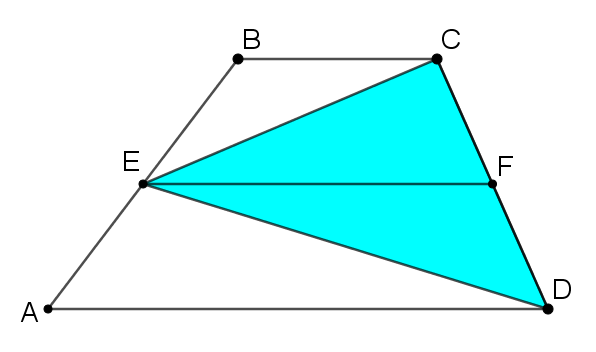How could you use this diagram to show that the area of the triangle is half the area of the trapezium?

Starting Point 2

Take a look at this sequence of three images. What happens at each stage?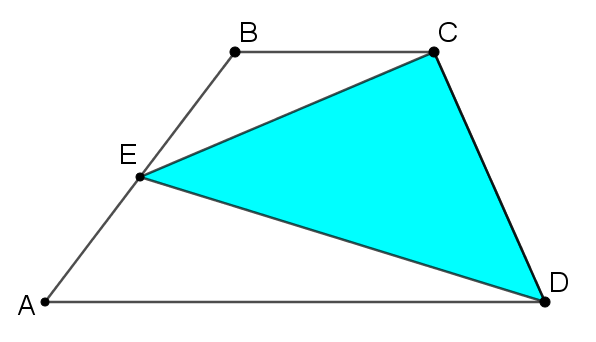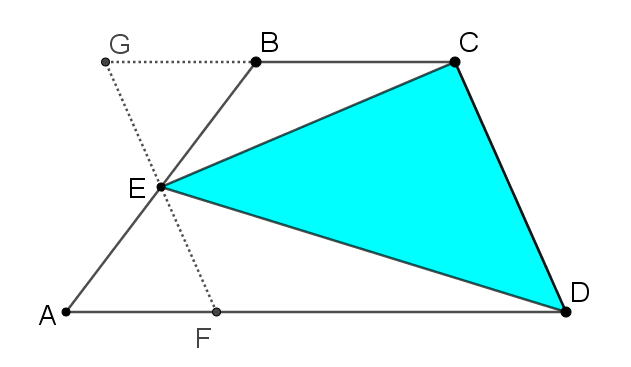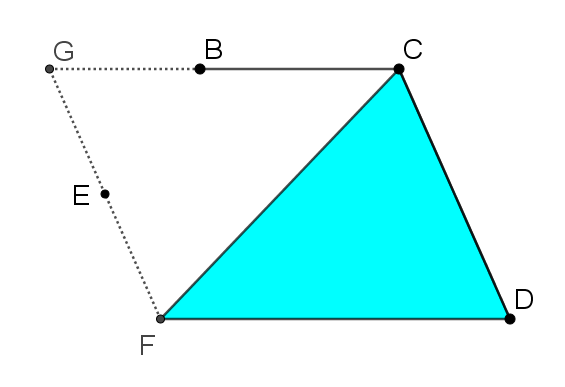How could you use this sequence of three images to create a proof that the area of the triangle is half the area of the trapezium?

Starting Point 3

Take a look at the image below. Can you see how the trapezium ABCD has been transformed to create this image?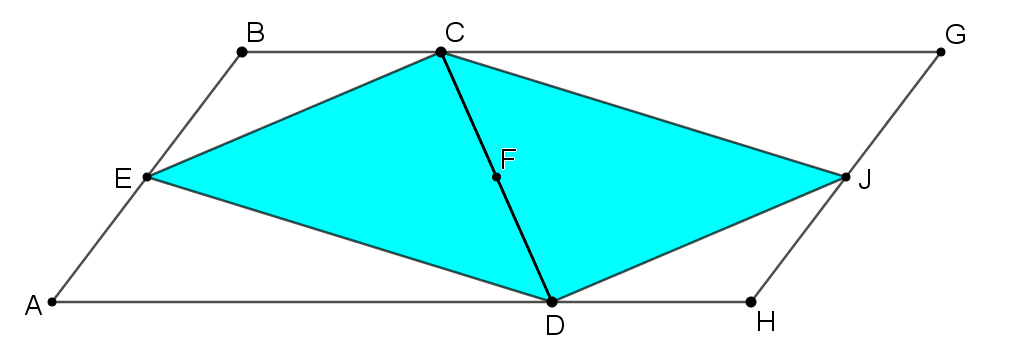How could you use this image to prove that Triangle CED has half the area of Trapezium ABCD? Click below to show two more images that might help.